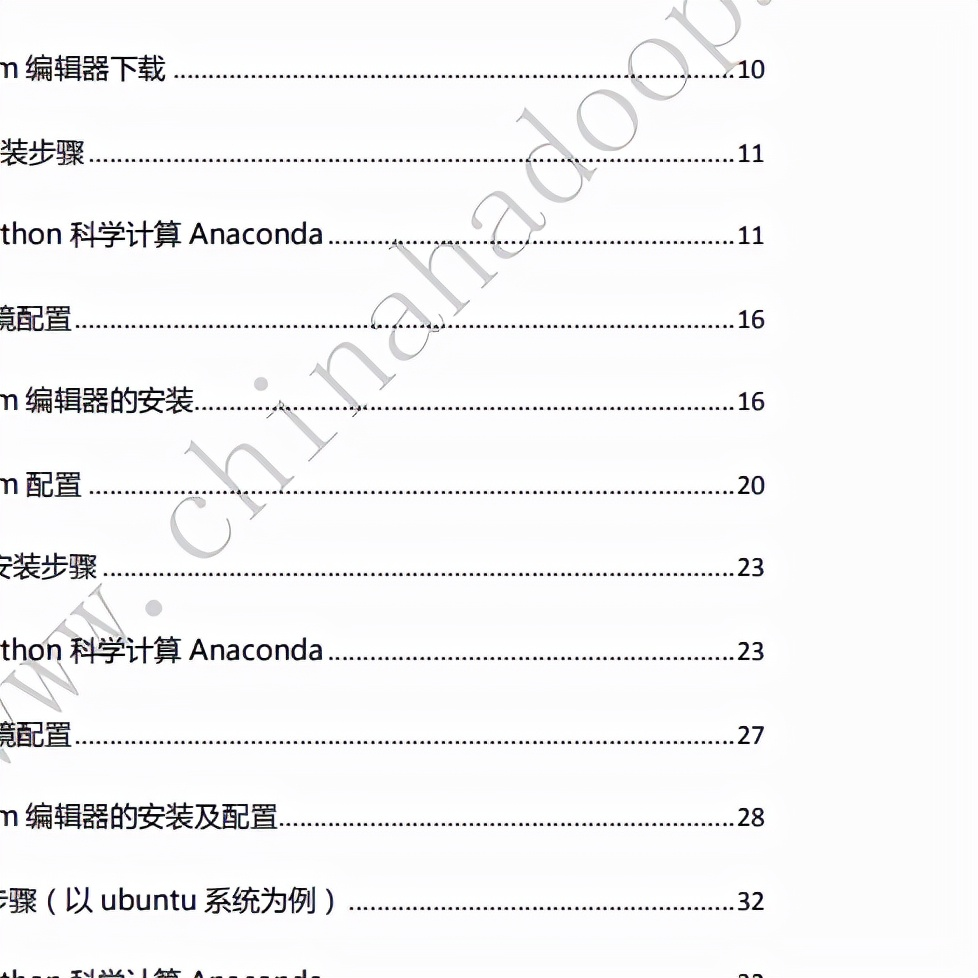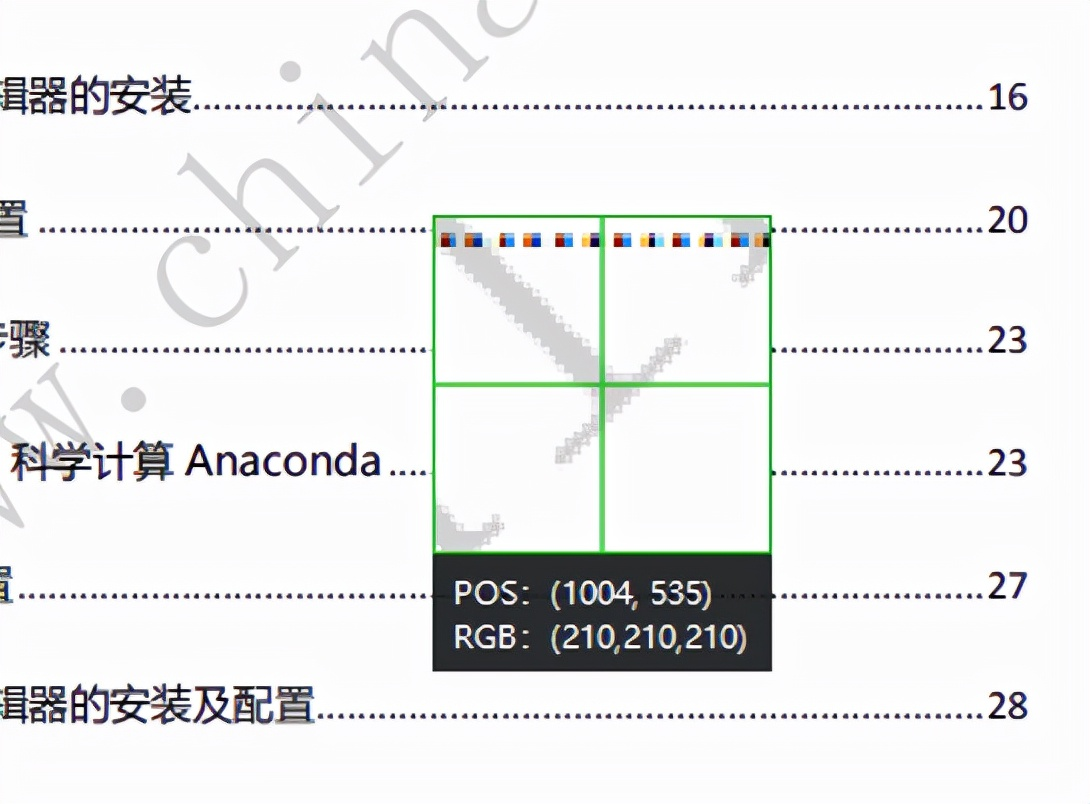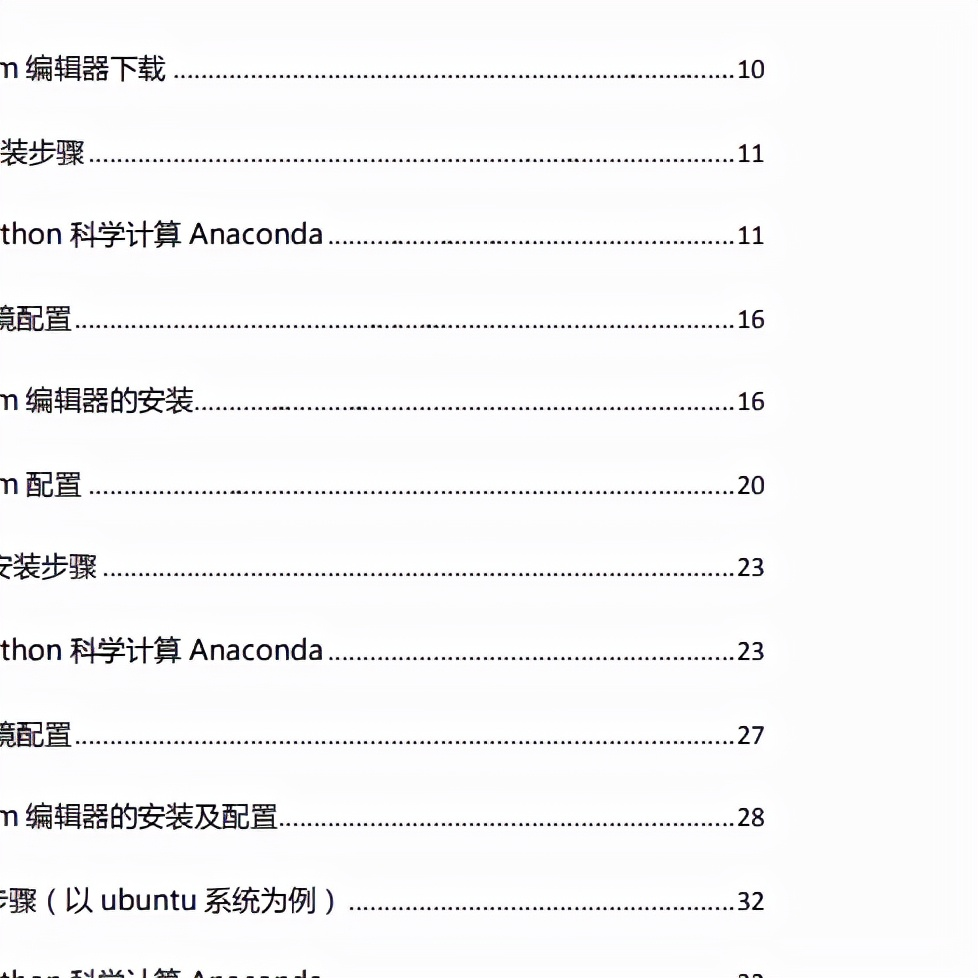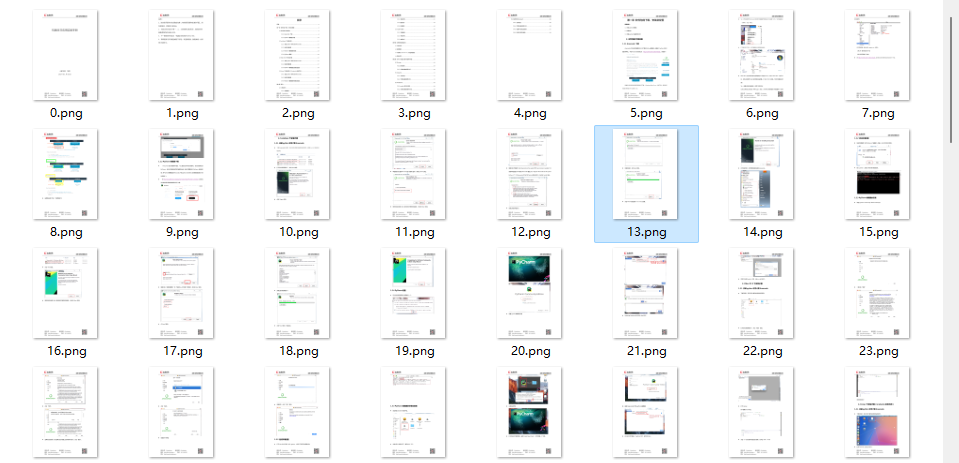##全国校区# 超简单！为图片和 PDF 上去掉水印（CDA干货分享）2022-01-17# 安装模块

PIL：Python Imaging Library 是 python 上非常强大的图像处理标准库，但是只能支持 python 2.7，于是就有志愿者在 PIL 的基础上创建了支持 python 3的 pillow，并加入了一些新的特性。

`pip install pillow`

pymupdf 可以用 python 访问扩展名为*.pdf、.xps、.oxps、.epub、.cbz或*.fb2的文件。还支持了许多流行的图像格式，包括多页TIFF图像。

`pip install PyMuPDF`

```from PIL import Image
from itertools import product import fitz import os```

# 获取图片的 RGB

pdf 去水印的原理和图片去水印的原理差不多，小编先从去除上面那张图片的水印开始。

`def remove_img(): image_file = input("请输入图片地址：") img = Image.open(image_file) width, height = img.size for pos in product(range(width), range(height)): rgb = img.getpixel(pos)[:3] print(rgb) `

# 图片去水印```rgb = img.getpixel(pos)[:3] if(sum(rgb) >= 620):
img.putpixel(pos, (255, 255, 255))

img.save('d:/qsy.png')```# PDF 去水印

PDF 去水印的原理和图片去水印的原理大致相同，用 PyMuPDF 打开 pdf 文件后，将 pdf 的每一页都转换为图片 pixmap，pixmap 有它自己的 RGB，只需要将 pdf 水印中的 RGB 改为(255, 255, 255) 最后保存为图片。

```def remove_pdf():
page_num = 0 pdf_file = input("请输入 pdf 地址：")
pdf = fitz.open(pdf_file); for page in pdf:
pixmap = page.get_pixmap() for pos in product(range(pixmap.width), range(pixmap.height)):
rgb = pixmap.pixel(pos, pos) if(sum(rgb) >= 620):
pixmap.set_pixel(pos, pos, (255, 255, 255))
pixmap.pil_save(f"d:/pdf_images/{page_num}.png") print(f"第{page_num}水印去除完成")
page_num = page_num + 1 ```# 图片转为 pdf

```def pic2pdf():
pic_dir = input("请输入图片文件夹路径：")

pdf = fitz.open()
img_files = sorted(os.listdir(pic_dir),key=lambda x:int(str(x).split('.'))) for img in img_files: print(img)
imgdoc = fitz.open(pic_dir + '/' + img)
pdfbytes = imgdoc.convertToPDF()
imgpdf = fitz.open("pdf", pdfbytes)
pdf.insertPDF(imgpdf)
pdf.save("d:/demo.pdf")
pdf.close()```

# 总结

pdf 和图片上恼人的水印终于可以在强大的 python 面前消失了。小伙伴们学会了吗？OK